# Plums

In the bowl are plums.

How many would be there if we can divide it equally among 8, 10 and 11 children?

x =  440

### Step-by-step explanation:Did you find an error or inaccuracy? Feel free to write us. Thank you!Tips to related online calculators
Do you want to calculate least common multiple two or more numbers?
Do you want to perform natural numbers division - find the quotient and remainder?

## Related math problems and questions:

• CherriesCherries in the bowl can be divided equally among 19 or 13 or 28 children. How many are the minimum cherries in the bowl?
• Nuts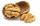How many we must have at least nuts if we can equally divide it to 10 children, 12 children or 15 children and any nut left?
• LCMWhat is the least common multiple of 5, 50, 14?
• Plum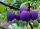On the platter are plum. How many were there if its have to be able to share equally among 8,10 and 12 children?
• The King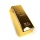The King wants to divide his sons equally. He has 42 rubies and 45 diamonds. How many sons and how will they share them?
• Lcm 2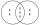Create the smallest possible number that is divisible by numbers 5,8,9,4,3
• GroupA group of kids wanted to ride. When the children were divided into groups of 3 children, one remain. When divided into groups of 4 children, 1 remains. When divided into groups of 6 children, 1 remains. After divided into groups of 5 children, no one lef
• LCM of two numberFind the smallest multiple of 63 and 147
• Find unknown numberWhat is the number between 50 and 55 that is divisible by 2,3,6,9?
• LCMCommon multiple of three numbers is 3276. One number is in this number 63 times, second 7 times, third 9 times. What are the numbers?
• Game roomWinner can took win in three types of jettons with value 3, 30 and 100 dollars. What is minimal value of win payable in this values of jettons?
• Lcm of three numbersWhat is the Lcm of 120 15 and 5
• Summer camp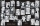Some boys or girls signed up for the summer camp, which has a maximum capacity of 200 children. The main leader noticed that during the evening start, he could arrange the participants exactly in the twelve-step, sixteen-step, or eighteen-step, and no one
• Trams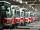Tram no. 3,7,10,11 rode together from the depot at 5am. Tram No. 3 returns after 2 hours, tram No. 7 an hour and half, no. 10 in 45 minutes and no. 11 in 30 minutes. For how many minutes and when these trams meet again?
• LCD 2The least common denominator of 2/5, 1/2, and 3/4
• Lesson exercising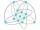The lesson of physical education, pupils are first divided into three groups so that each has the same number. The they redistributed, but into six groups. And again, it was the same number of children in each group. Finally they divided into nine equal g
• On Children'sOn Children's Day, the organizers bought 252 chewing gums, 396 candies and 108 lollipops. They want to make as many of the same packages as possible. Advise them what to put in each package and how many packages they can make this way.